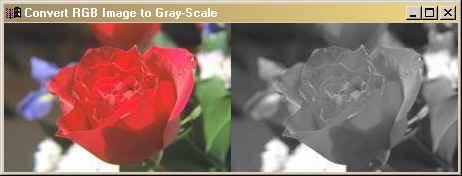# Convert RGB Image to Grayscale

QUESTION: How do I convert a color image (say a JPEG image) to grayscale?ANSWER: This answer from Craig Markwart on the IDL newsgroup:

There is no one "correct" conversion from RGB to grayscale, since it depends on the sensitivity response curve of your detector to light as a function of wavelength. A common one in use is:
```    Y = 0.3*R + 0.59*G + 0.11*B
```

Using Craig's formula, then, here is an example:

```   filename = Filepath(Subdir=['examples', 'data'], 'rose.jpg')
Read_JPEG, filename, rose
rose_gray = 0.3*Reform(rose[0,*,*]) + 0.59*Reform(rose[1,*,*]) + 0.11*Reform(rose[2,*,*])
```

Here is the result.Web Coyote's Guide to IDL Programming